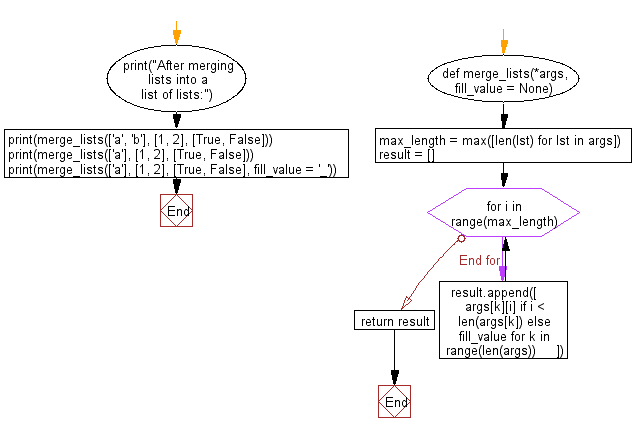﻿ Python: Merge two or more lists into a list of lists - w3resource# Python: Merge two or more lists into a list of lists

## Python List: Exercise - 215 with Solution

Write a Python program to merge two or more lists into a list of lists, combining elements from each of the input lists based on their positions.

Merges two or more lists into a list of lists, combining elements from each of the input lists based on their positions.

• Use max() combined with a list comprehension to get the length of the longest list in the arguments.
• Use range() in combination with the max_length variable to loop as many times as there are elements in the longest list
• If a list is shorter than max_length, use fill_value for the remaining items (defaults to None)
• zip() and itertools.zip_longest() provide similar functionality to this snippet

Sample Solution:

Python Code:

``````def merge_lists(*args, fill_value = None):
max_length = max([len(lst) for lst in args])
result = []
for i in range(max_length):
result.append([
args[k][i] if i < len(args[k]) else fill_value for k in range(len(args))
])
return result
print("After merging lists into a list of lists:")
print(merge_lists(['a', 'b'], [1, 2], [True, False]))
print(merge_lists(['a'], [1, 2], [True, False]))
print(merge_lists(['a'], [1, 2], [True, False], fill_value = '_'))
```
```

Sample Output:

```After merging lists into a list of lists:
[['a', 1, True], ['b', 2, False]]
[['a', 1, True], [None, 2, False]]
[['a', 1, True], ['_', 2, False]]
```

Flowchart:## Visualize Python code execution:

The following tool visualize what the computer is doing step-by-step as it executes the said program:

Python Code Editor:

Have another way to solve this solution? Contribute your code (and comments) through Disqus.

What is the difficulty level of this exercise?

Test your Python skills with w3resource's quiz

﻿

## Python: Tips of the Day

```print(2_000_000)
```2000000